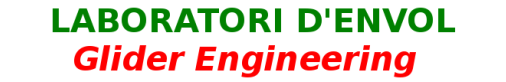REFLEXCREATOR

Mathematical procedure to provide reflex to any profile. Attached spreadsheet, and theoretical explanation.

It is a very simple geometrical procedure, this is to deform the back of the profile, raising the trailing edge smoothly, with a parabolic function, as explained in the figure.

If (X,Y) are the original airfoil coordinates, then the correction in "Y" is:

Y'=Y     if X< Xo
Y'=Y + (b/a^2)*(X-Xo)^2     if X >=Xo
X'=X

where (X',Y') are the new reflexed coordinates

It is very important export coordinates to a .dat file (making sure the  decimal point is "." not ","), and then apply the function "derotate" and "normalize" with XFLR5 or XFOIL.

The difficulty lies in determining if the resulting profile is really "good."  To do this, we must carefully analyze the parameters Cl, Cx, Cm with variations in angle of attack, and compared with other known profiles.  Interestingly, any profile can become slightly or strongly reflex, by  applying this simple transformation.